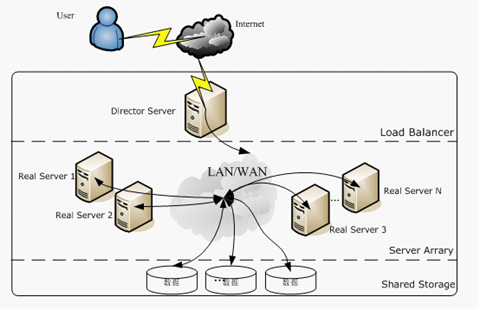# 推荐算法之矩阵分解

## 矩阵分解简介

### 矩阵分解的基本思路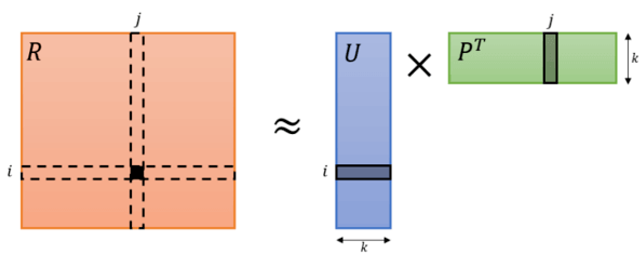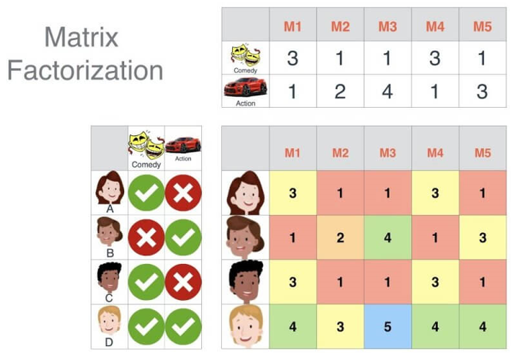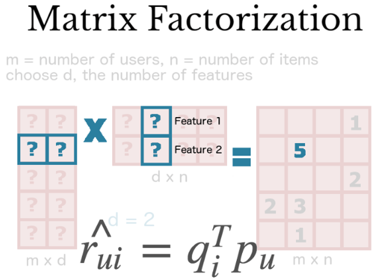### 矩阵分解的优缺点

• 比较容易编程实现，随机梯度下降方法依次迭代即可训练出模型。比较低的时间和空间复杂度，高维矩阵映射为两个低维矩阵节省了存储空间，训练过程比较费时，但是可以离线完成；评分预测一般在线计算，直接使用离线训练得到的参数，可以实时推荐。
• 预测的精度比较高，预测准确率要高于基于领域的协同过滤以及内容过滤等方法。
• 非常好的扩展性，很方便在用户特征向量和物品特征向量中添加其它因素，例如添加隐性反馈因素的SVD++，此方法的详细实现参见文献《Koren Y. Factorization meets the neighborhood: a multifaceted collaborative filtering model》；添加时间动态time SVD++，此方法将偏置部分和用户兴趣都表示成一个关于时间的函数，可以很好的捕捉到用户的兴趣漂移，欲知详细实现请阅读文献《Koren Y. Collaborative filtering with temporal dynamics》。

• 模型训练比较费时。
• 推荐结果不具有很好的可解释性，分解出来的用户和物品矩阵的每个维度无法和现实生活中的概念来解释，无法用现实概念给每个维度命名，只能理解为潜在语义空间。

## 矩阵分解相关算法

### 传统的SVD算法

$$A_{m\times n} \approx U_{m\times k} \Sigma _{k \times k}V_{k \times n}^T$$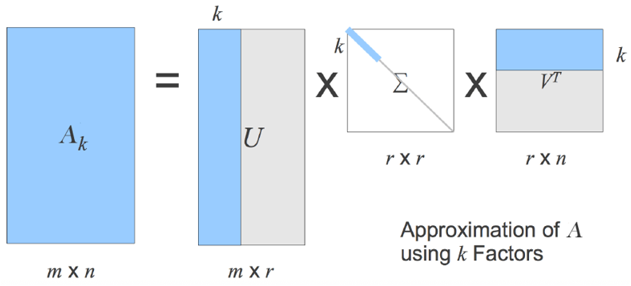• SVD 分解要求矩阵是稠密的，而现实场景中的评分矩阵是稀疏的，有大量空白，无法直接使用 SVD 分解的。要想使用 SVD，必须对评分矩阵中的缺失值进行简单的补全，比如用全局平均值或者用用户物品平均值补全，得到补全后的矩阵。接着可以用 SVD 分解并降维。但填充本身会造成很多问题，其一，填充大大增加了数据量，增加了算法复杂度。其二，简单粗暴的数据填充很容易造成数据失真。
• SVD 分解的复杂度比较高，假设对一个$m \times n$的矩阵进行分解，时间复杂度为$O(n^2∗m+n∗m^2)$，其实就是$O(n^3)$。对于 m、n 比较小的情况，还是勉强可以接受的，但是在推荐场景的海量数据下，m 和 n 的值通常会比较大，可能是百万级别上的数据，这个时候如果再进行 SVD 分解需要的计算代价就是很大的。

### Funk-SVDSimon Funk 在博客上公开发表了一个只考虑已有评分记录的矩阵分解方法，称为 Funk-SVD，也就是被 Yehuda Koren 称为隐语义模型的矩阵分解方法。它的出发点为，既然将一个矩阵做 SVD 分解成 3 个矩阵很耗时，同时还面临稀疏的问题，那么我们能不能避开稀疏问题，同时只分解成两个矩阵呢？也就是说，现在期望我们的矩阵M这样进行分解：

$$M_{m\times n}=P^T_{m\times k}Q_{k\times n}$$

$\sum_{i，j}(m_{ij}-q^T_jp_i)^2$

$$arg \underset {p_jq_j}{min}\sum_{(i,j)\in K}(m_{ij}-q_j^Tp_i)^2+\lambda (||p_i||^2_2+||q_j||^2_2)$$

$$\frac{\partial J}{\partial p_i} = -2(m_{ij}-q_j^Tp_i)q_j + 2\lambda p_i$$

$$\frac{\partial J}{\partial q_j} = -2(m_{ij}-q_j^Tp_i)p_i + 2\lambda q_j$$

$$p_i = p_i + \alpha((m_{ij}-q_j^Tp_i)q_j – \lambda p_i)$$

$$q_j =q_j + \alpha((m_{ij}-q_j^Tp_i)p_i – \lambda q_j)$$

### Bias-SVD

Funk-SVD方法通过学习用户和物品的特征向量进行预测，即用户和物品的交互信息。用户的特征向量代表了用户的兴趣，物品的特征向量代表了物品的特点，且每一个维度相互对应，两个向量的内积表示用户对该物品的喜好程度。但是我们观测到的评分数据大部分都是都是和用户或物品无关的因素产生的效果，即有很大一部分因素是和用户对物品的喜好无关而只取决于用户或物品本身特性的。例如，对于乐观的用户来说，它的评分行为普遍偏高，而对批判性用户来说，他的评分记录普遍偏低，即使他们对同一物品的评分相同，但是他们对该物品的喜好程度却并不一样。同理，对物品来说，以电影为例，受大众欢迎的电影得到的评分普遍偏高，而一些烂片的评分普遍偏低，这些因素都是独立于用户或产品的因素，而和用户对产品的的喜好无关。

• 训练集中所有评分记录的全局平均数$\mu$，表示了训练数据的总体评分情况，对于固定的数据集，它是一个常数。
• 用户偏置$b_i$，独立于物品特征的因素，表示某一特定用户的打分习惯。例如，对于批判性用户对于自己的评分比较苛刻，倾向于打低分；而乐观型用户则打分比较保守，总体打分要偏高。
• 物品偏置$b_j$，特立于用户兴趣的因素，表示某一特定物品得到的打分情况。以电影为例，好片获得的总体评分偏高，而烂片获得的评分普遍偏低，物品偏置捕获的就是这样的特征。

$$\underbrace{arg\;min}_{p_i,q_j}\;\sum\limits_{i,j}(m_{ij}-\mu-b_i-b_j-q_j^Tp_i)^2 + \lambda(||p_i||_2^2 + ||q_j||_2^2 + ||b_i||_2^2 + ||b_j||_2^2)$$

$$b_i = b_i + \alpha(m_{ij}-\mu-b_i-b_j-q_j^Tp_i -\lambda b_i)$$

$$b_j = b_j + \alpha(m_{ij}-\mu-b_i-b_j-q_j^Tp_i -\lambda b_j)$$

Bias-SVD的理解：

• 在FunkSVD的基础上加入了Baseline Predictors.
• 为什么要加入：user_i对item_j的评分是3分，如果mean_rate是2，那么3分就很高。如果mean_rate是4.7，那么3分就很低。

### SVD++

SVD++是对 BiasSVD 的进一步改进，引入了隐式反馈和用户属性的信息，相当于引入了额外的信息源，这样可以从侧面反映用户的偏好，而且能够解决因显式评分行为较少导致的冷启动问题。

$$\underbrace{arg\;min}_{p_i,q_j}\;\sum\limits_{i,j}(m_{ij}-\mu-b_i-b_j-q_j^Tp_i – q_j^T|N(i)|^{-1/2}\sum\limits_{s \in N(i)}y_{s})^2+ \lambda(||p_i||_2^2 + ||q_j||_2^2 + ||b_i||_2^2 + ||b_j||_2^2 + \sum\limits_{s \in N(i)}||y_{s}||_2^2)$$

• 用户i对物品j的兴趣程度 = 用户i对物品j的显式反馈(大概率缺失) + 用户i的一系列隐式反馈在物品j上表现；
• 定义一组关于item的隐式反馈特征：比如用户u对每一个item的浏览时间；因为是关于item的特征，所以保证了长度和item一样。
• $|N(u)|$表示用户u在隐式反馈特征中有行为的个数。
• $|y_i|$表示用户u在隐式反馈上的表对将要评分item的隐性影响的权重数。可以理解为一种行为的数值化，如果没有就用0填充，保证长度一致；

• 隐式反馈特征定义为：用户浏览过电影的电影介绍页的停留时间。
• 用户u在这个隐式反馈特征上的表现必定不一样。故$|N(u)|$长度不一样。使用$1/(|N(u)|)^-(1/2)$来平衡个数不同带来的影响。这种标准化的方法纯属是经验，无理论根据。
• 举个例子：如果用户u对电影i有评分，且已知其在电影介绍页的停留时间，那么这种“修正方式”更能体现用户u对电影i的兴趣程度。
• 可以认为，SVD++是一种SVD基于特征组合的扩展，也是对SVD原始的latent factor的一种修正。

### TimeSVD++

$$\hat{r}_{ui} = \mu + b_u(t) + b_i(t) + q_i^Tp_u(t)$$

$$b_u(t) = b_u + \frac{\sum_{l=1}^{k_u} e^{-\gamma|t-t_l^u| } b_{t_l}^u}{\sum_{l=1}^{k_u} e^{-\gamma|t-t_l^u| } }$$

$$b_u(t) = b_u + \frac{\sum_{l=1}^{k_u} e^{-\gamma|t-t_l^u| } b_{t_l}^u}{\sum_{l=1}^{k_u} e^{-\gamma|t-t_l^u| } } + b_{u, period(t)}$$

• 出发点：它的最后一列是用户作出该评价的时间，timeSVD++就是将时间这个信息加以了利用，比较直观的理解就是影片的受欢迎程度可能是随着时间的变化而变化的，某些电影可能还具有周期性。
• 玩法很简单，使用户过去的行为成为一个随时间衰减的变量，比如可以用Adam中用过的指数衰减法“EWMA(Exponentially Weighted Moving Average，指数加权移动平均) ”
• timeSVD++其实是衰减过往用户行为的权重，提高就近用户隐式反馈行为的权重。

### NMF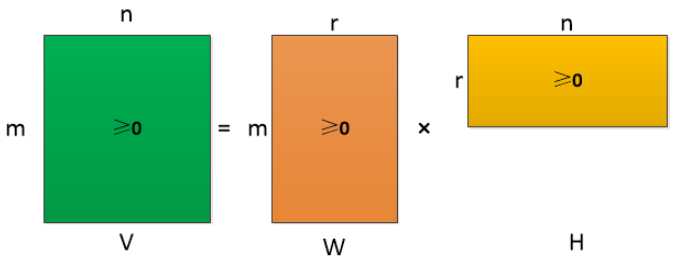NMF中要求原始的矩阵V的所有元素的均是非负的，那么矩阵V可以分解为两个更小的非负矩阵的乘积，这个矩阵V有且仅有一个这样的分解，即满足存在性和唯一性。

$$L\left ( \mathbf{W}, \mathbf{H} \right ) = \frac{1}{2} \left | \mathbf{R} – \mathbf{W}\mathbf{H^T} \right | + \frac{\lambda}{2}\left ( \left | \mathbf{W} \right |_F^2 + \left | \mathbf{H} \right |_F^2 \right )$$

$$L\left ( \mathbf{W}, \mathbf{H} \right ) = \frac{1}{2} \sum_{i = 1}^{m}\sum_{j = 1}^{n}\left ( r_{i,j} – w_i h_j^T \right ) + \frac{\lambda}{2}\left ( \left | \mathbf{W} \right |_F^2 + \left | \mathbf{H} \right |_F^2 \right )$$

$$\frac{\partial L}{\partial w_i} = -\sum_{j = 1}^{n}(r_{i,j} – w_i h_j^T)h_j + \lambda w_i = G_{w_i} + F_{w_i}$$

$$\frac{\partial L}{\partial h_j} = -\sum_{i = 1}^{m}(r_{i,j} – w_i h_j^T)w_i + \lambda h_j = G_{h_j} + F_{h_j}$$

$$G_{w_i} = -\sum_{j = 1}^{n}(r_{i,j} – w_i h_j^T)h_j$$

$$F_{w_i} = \lambda w_i$$

$$G_{h_j} = -\sum_{i = 1}^{m}(r_{i,j} – w_i h_j^T)w_i$$

$$F_{h_j} = \lambda h_j$$

$$w_i \leftarrow \left [ (1 – \eta \lambda)w_i + \eta \sum_{j = 1}^{n}e_{i,j}h_j \right ]_+$$

$$h_j \leftarrow \left [ (1 – \eta \lambda)h_j + \eta \sum_{i = 1}^{m}e_{i,j}w_i \right ]_+$$

$$W = DenseMatrix(m, K) \times \sqrt{\frac{\mu}{K}}$$

$$H = DenseMatrix(K, n) \times \sqrt{\frac{\mu}{K}}$$

$$\text{learnrate} = \frac{lr} { math.sqrt(step)}$$

$$p_{uf} \leftarrow p_{uf} \cdot \frac{\sum_{i \in I_u} q_{if} \cdot r_{ui}}{\sum_{i \in I_u} q_{if} \cdot \hat{r_{ui}} + \lambda_u |I_u| p_{uf}}$$

$$q_{if} \leftarrow q_{if} \cdot \frac{\sum_{u \in U_i} p_{uf} \cdot r_{ui}}{\sum_{u \in U_i} p_{uf} \cdot \hat{r_{ui}} + \lambda_i |U_i| q_{if}}$$

### WRMF

WRMF 的目标函数：

$$\underset {p^*,q^*}{\min} \sum_{r_{ui} \in R_{O} \cup R_{-O}} c_{ui}\left(r_{ui} – p_u^Tq_i \right)^2 + \lambda\left(||q_i||^2 + ||p_u||^2\right)$$

$$c_{ui}=1+\alpha C_{ui}$$

• 该损失也可以通过下个算法 PMF 推导，此时$c_{ui}^{-1}$即为 PMF 中评分$r_{ij} \sim N(p_u^Tq_i, c_{ui}^{-1})$的方差。$c_{ui}$越大，方差越小，置信值越高。
• 这种加权的方法除了可用于隐式反馈，还可以根据某些短期策略或因素的影响进行加权，比如一些短时间大规模的广告会影响一部分物品的隐式反馈，而这并不能恰当地反应长期的特点。

One-Class 的负样本如何采样？

• 随机均匀采样和正类别一样多；
• 按照物品的热门程度采样。

### PMF

PMF，即概率矩阵分解（Probabilistic Matrix Factorization）。设有 N 个用户和 M 个商品，评分系统用$N \times M$的矩阵 R 表示。基本思路：仍然是矩阵的低秩近似。即$R=U^T V$，用户和商品之间的关系（即用户对商品的偏好）可以由较少的几个因素的线性组合决定。这也是 MF 的基本思想。PMF在MF 的基础上加上了贝叶斯观点，即认为R是观测到的值，U、V 描述了系统的内部特征，是需要估计的。

• 对一个近似矩阵进行分解$\hat{R} =U^TV$
• 要求近似矩阵$\hat{R}$在观测到的评分部分和观测矩阵R尽量相似
• 为了防止过拟合，需要对 U、V 做某种形式的约束

• 观测噪声（观测评分矩阵 R 和近似评分矩阵$\hat{R}$之差）为高斯分布。
• 用户属性 U 和商品属性 V 均为高斯分布。

$$P(R_{ij}-U_i^TV_j) \sim N(0,\sigma^2), \\ denotes: P(R_{ij}-U_i^TV_j |0,\sigma^2)$$

$$P(R|U,V,\sigma^2)=\prod_{i=1}^N\prod_{j=1}^M[N(R_{ij}|U_i^TV_j,\sigma^2)]^{I_{ij}}$$

$$p(U|\sigma^2_U)=\prod_{i=1}^N N(U_i|0,\sigma^2_UI) \\ p(V|\sigma^2_V)=\prod_{i=1}^M N(V_i|0, \sigma^2_V I)$$

$$P(U,V|R,\sigma^2,\sigma_U^2,\sigma_V^2)=P(R|U,V,\sigma^2)P(U|\sigma_U^2)P(V|\sigma_V^2)$$

$$lnP(U,V|R,\sigma^2,\sigma_U^2,\sigma_V^2) = \\ -\frac{1}{2\sigma^2}\sum_{i=1}^N \sum_{j=1}^M I_{ij}(R_{ij}-U_i^TV_j)^2\\ -\frac{1}{2\sigma_U^2}\sum_{i=1}^N U_i^T U_i \\ – \frac{1}{2\sigma_V^2}\sum_{j=1}^M V_j^T V_j \\ -\frac{1}{2}\left (\left( \sum_{i=1}^N \sum_{j=1}^M I_{ij}\right) ln \sigma^2 + ND\ln \sigma_U^2 + MD \ln \sigma_V^2 \right)\\ +C$$

$$E =\frac{1}{2}\sum_{i=1}^N \sum_{j=1}^M I_{ij}(R_{ij}-U_i^TV_j)^2 + \frac{\lambda_U}{2}\sum_{i=1}^N ||U_i||_F^2 + \frac{\lambda_V}{2}\sum_{j=1}^M ||V_j||_F^2 \\ \lambda_U=\frac{\sigma^2}{\sigma_U^2} , \lambda_V=\frac{\sigma^2}{\sigma_V^2}$$

$$E(U,V)=\frac{1}{2}\sum_{ij}I_{ij}(R_{ij}-g(U_i^TV_j))^2+\frac{\lambda_U}{2}\sum_iU_i^TU_i + \frac{\lambda_V}{2}\sum_jV_j^TV_j$$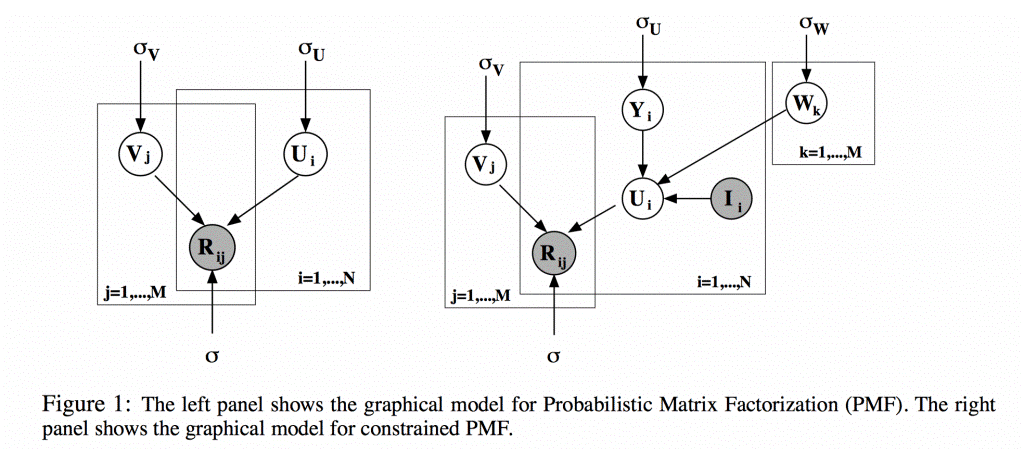$$U_i = Y_i + \frac{1}{\sum_kI_{ik}}\sum_kI_{ik}W_k$$

### ALS

$$R_{m \times n}=P_{m \times k} \times Q_{n \times k}^T$$

$$P_{m \times k}=R_{m \times n} \times Q_{n \times k}^{-1}$$

• 初始化随机矩阵 Q 里面的元素值
• 把 Q 矩阵当做已知的，直接用线性代数的方法求得矩阵 P
• 得到了矩阵 P 后，把 P 当做已知的，故技重施，回去求解矩阵 Q
• 上面两个过程交替进行，一直到误差可以接受为止

## 矩阵分解高级演化

### Embedding

Embedding 在推荐中的一些用法：

• 获得用户和物品的隐语义表达
• 作为深度神经网络的输入
• 根据物品映射得到的特征向量去找相似的物品

Embedding 本质上也是体现物品关联，但是比协同过滤的覆盖度高。由此，Embedding 几乎成为高级推荐算法的必用技术，Embedding 的特征预处理可以带来更好的效果，又能降维又能挖掘深层关联信息，何乐而不为呢？与矩阵分解类似，其核心思想就是同时构建用户和物品的嵌入式表示，使得多种实体的嵌入式表示存在于同一个隐含空间内， 进而挖掘实体之间的关联信息 (非端到端)，以及实体和最终目标任务之间的关联信息（端到端）。

### 分解机FM

$$y(\mathbf{x}) = w_0+ \sum_{i=1}^n w_i x_i + \sum_{i=1}^n \sum_{j=i+1}^n w_{ij} x_i x_j$$

$x_i$、$x_j$是经过One-hot编码后的特征，取 0 或 1。只有当二者都为1时，$w_{ij}$权重才能得以学习。然后由于稀疏性的存在，满足$x_i$，$x_j$都非零的样本很少，导致组合特征权重参数缺乏足够多的样本进行学习。

FM模型公式

$$y(\mathbf{x}) = w_0+ \sum_{i=1}^n w_i x_i + \sum_{i=1}^n \sum_{j=i+1}^n \langle \mathbf{v}_i, \mathbf{v}_j \rangle x_i x_j$$

$$\left \langle v_i,v_j \right \rangle=\sum_{f=1}^kv_{i,f}\cdot v_{j,f}$$

• 线性模型+交叉项：直观地看 FM 模型表达式，前两项是线性回归模型的表达式，最后一项是二阶特征交叉项（又称组合特征项），表示模型将两个互异的特征分量之间的关联信息考虑进来。用交叉项表示组合特征，从而建立特征与结果之间的非线性关系。
• 交叉项系数→隐向量内积：由于FM 模型是在线性回归基础上加入了特征交叉项，模型求解时不直接求特征交叉项的系数$w_{ij}$（因为对应的组合特征数据稀疏，参数学习不充分），故而采用隐向量的内积$w_{ij}=\left \langle v_i,v_j \right \rangle$表示$w_{ij}$。具体的，FM 求解过程中的做法是：对每一个特征分量$x_i$引入隐向量$v_i＝(v_{i,1},v_{i,2},⋯,v_{i,k})$，利用$v_iv_j^T$ 内积结果对交叉项的系数$w_{ij}$进行估计，公式表示：$\hat {w}_{ij}=v_iv^T_j$。

$$\left \langle v_h,v_i \right \rangle=\sum_{f=1}^k v_{h,f} \cdot v_{i,f}$$

$$\left \langle v_i,v_j \right \rangle=\sum_{f=1}^k v_{i,f} \cdot v_{j,f}$$

$x_hx_i$与$x_ix_j$的系数分别为$\left \langle v_h,v_i \right \rangle$和$\left \langle v_i,v_j \right \rangle$，它们之间有共同项$v_i$，也就是说，除了$x_i$自身，所有包含$x_i$的非零组合特征（存在某个$j\neq i$, 使得$x_ix_j\neq 0$）的样本也都可以用来学习隐向量$v_i$，这在很大程度上避免了数据稀疏性造成参数估计不准确的影响。而在多项式模型中，$w_{hi}$和$w_{ij}$是相互独立的。

FM 模型复杂度

FM 模型公式直观来看，时间复杂度为$O(n^2)$，n是特征的数量，但是，通过下面的等价转换，可以将 FM 的二次项化简，其复杂度可以优化到$O(kn)$，即：

\begin{aligned} \sum_{i=1}^{n-1}\sum_{j=i+1}^n(\boldsymbol{v}_i^T \boldsymbol{v}_j)x_ix_j &= \frac{1}{2}\left(\sum_{i=1}^n\sum_{j=1}^n(\boldsymbol{v}_i^T \boldsymbol{v}_j)x_ix_j-\sum_{i=1}^n(\boldsymbol{v}_i^T \boldsymbol{v}_i)x_ix_i\right)\\ &=\frac{1}{2}\left(\sum_{i=1}^n\sum_{j=1}^n\sum_{l=1}^kv_{il}v_{jl}x_ix_j-\sum_{i=1}^n\sum_{l=1}^k v_{il}^2x_i^2\right)\\\ &=\frac{1}{2}\sum_{l=1}^k\left(\sum_{i=1}^n(v_{il}x_i)\sum_{j=1}^n(v_{jl}x_j)-\sum_{i=1}^nv_{il}^2x_i^2\right)\\ &=\frac{1}{2}\sum_{l=1}^k\left(\left(\sum_{i=1}^n(v_{il}x_i)\right)^2-\sum_{i=1}^n (v_{il}x_i)^2\right)\\ \end{aligned}

FM 模型的优化

$$\frac{\partial}{\partial\theta}y\left(x\right)=\begin{cases} 1, & if \ \theta\ is\ w_0\\ x_i,\ & if\ \theta\ is\ w_i\\ x_i\sum_{j=1}^n v_{j,f}x_j-v_{i,f}x_{i}^{2},\ & if\ \theta\ is\ v_{i,f}\\ \end{cases}$$

FM 的模型可用的损失函数

 FM 应用场景 损失函数 说明 回归 均方误差（MSE）损失 Mean Square Error，与平方误差类似 二类分类 Hinge/Cross-Entopy 损失 分类时，结果需要做 sigmoid 变换 排序

FM 模型对稀疏数据有更好的学习能力，通过交互项可以学习特征之间的关联关系，并且保证了学习效率和预估能力，在工业界使用非常多。

### 场感知分解机FFM

$$y(\mathbf{x}) = w_0 + \sum_{i=1}^n w_i x_i + \sum_{i=1}^n \sum_{j=i+1}^n \langle \mathbf{v}_{i, f_j}, \mathbf{v}_{j, f_i} \rangle x_i x_j$$

Yu-Chin Juan 实现了一个C++版的FFM 模型，即比较出名的 libffm，也有人在此之上开发出了 Python 接口。这个版本的 FFM 省略了常数项和一次项，模型方程如下：

$$\phi (w,x)=\sum_{j1,j2 \in C_2}⟨w_{j_1,f_2},w_{j_2,f_1}⟩x_{j_1}x_{j_2}$$

$$\underset{w}{min}\sum_{i=1}^L \log(1+\exp{−y_i \phi(w,x_i)})+\frac{λ}{2}‖w‖2$$

### 协同矩阵分解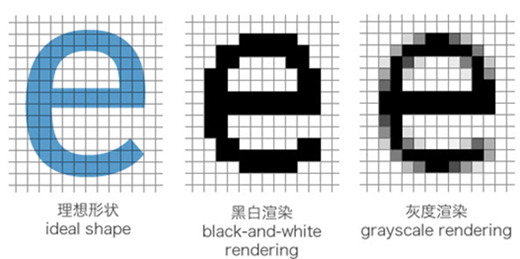##### 网站与APP开发中的字体设置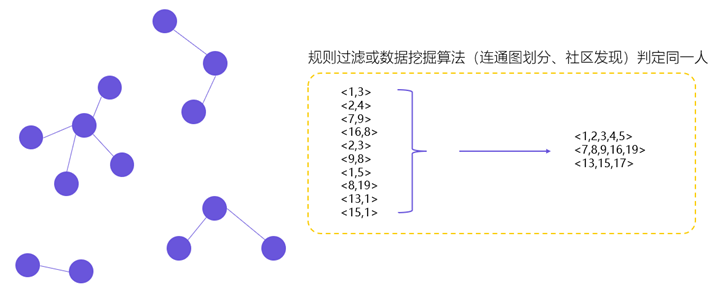##### 用户体系搭建之ID-Mapping